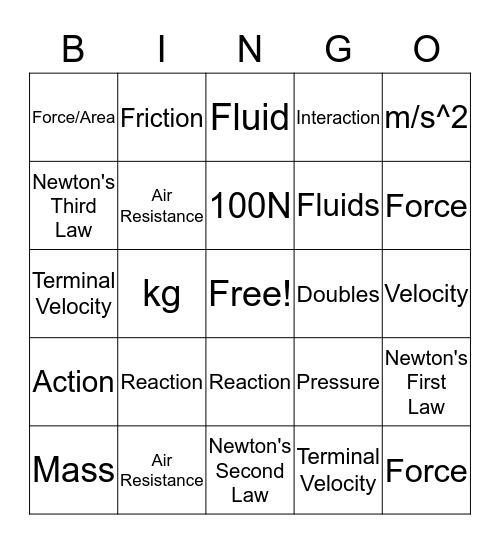# Physics BingoThis bingo card has a free space and 49 words: Acceleration, Force, Mass, Newton's Second Law, Reaction, Action, Friction, Net Force, Newton's Third Law, Terminal Speed, Air Resistance, Interaction, Newton's First Law, Pressure, Velocity, Increase, 100N, Doubles, Force/ Acceleration, m/s^2, kg, Terminal Velocity, Force/Area, Fluids, Acceleration, Force, Mass, Newton's Second Law, Reaction, Action, Friction, Net Force, Newton's Third Law, Terminal Speed, Air Resistance, Interaction, Newton's First Law, Pressure, Velocity, Newtons, Physics, Vector, m/s^2, kg, Terminal Velocity, Fluid, P=F/A, F=M*A and M=F/A.

⚠ This card has duplicate items: Acceleration (2), Force (2), Mass (2), Reaction (2), Action (2), Friction (2), Interaction (2), Pressure (2), Velocity (2), m/s^2 (2), kg (2)

## Play Online